# Vectors in Physics

## Introduction

• In previous chapter we have learned about the motion of any particle along a straight line
• Straight line motion or rectilinear motion is motion in one dimension.Now in this chapter ,we will consider both motion in two dimension and three dimension.
• In two dimensional motion path of the particle is constrained to lie in a fixed plane.Example of such motion motion are Projectile shot from a gun ,motion of moon around the earth,circular motion and many more.
• To solve problems of motion in a plane,we need to generalize kinematic language of previous chapter to a more general using vector notations in two and three dimensions.
• In the next section ,we will be learning about Scalar and Vectors, Vectors in Physics and two dimensional motion.

## Difference between Scalar and Vector

Physical quantities may be divided into two categories
(1) Scalars are physical quantities that only have magnitude for example mass, length, time, temperature ,the distance between two points, mass of an object, and the time at which a certain event happened. The rules for combining scalars are the rules of ordinary algebra. Scalars can be added, subtracted, multiplied and divided just as the ordinary numbers
(2)Vectors are physical quantities having both magnitude and direction for example velocity, force, electric field, torque etc. It can be represented by an arrow in space.It obeys the triangle law of addition or equivalently the parallelogram law of addition.

## What is vector

• A quantity that has magnitude as well as direction is called vector.From a geometric point of view, a vector can be defined as a line segment having a specific direction and a specific length
• It is denoted by the letter bold letter $\textbf{a}$ or it can be denoted as $\vec{a}$
• Magnitude of a vector a is denoted by |a| or a.It is a positive quantity
• It obeys the triangle law of addition or equivalently the parallelogram law of addition
• Example velocity, force, electric field, torque,acceleration etc

## Type Of Vectors

Zero or Null Vector :A vector whose initial and terminal points are coincident is called zero or null vector
Unit Vector :A vector whose magnitude is unity is called a unit vector which is denoted by n^
Free Vectors: If the initial point of a vector is not specified, then it is said to be a free vector.
Negative of a Vector A vector having the same magnitude as that of a given vector a and the direction opposite to that of a is called the negative of a and it is denoted by —a.
Like and Unlike Vectors Vectors are said to be like when they have the same direction and unlike when they have opposite direction.
Collinear or Parallel Vectors Vectors having the same or parallel supports are called collinear vectors.
Co-initial Vectors Vectors having same initial point are called co-initial vectors.
Coplanar Vectors A system of vectors is said to be coplanar, if their supports are parallel to the same plane. Otherwise they are called non-coplanar vectors
(Equal vectors: Two vectors a and b are said to be equal written as a = b, if they have  same magnitude and same direction regardless of the there initial point

Let a and b be any two vectors. From the terminal point of a, vector b is drawn. Then, the vector from the initial point  of a to the terminal point B of b is called the sum of vectors a and b and is denoted by a + b. This is called the triangle law of addition of vectors
c= a + b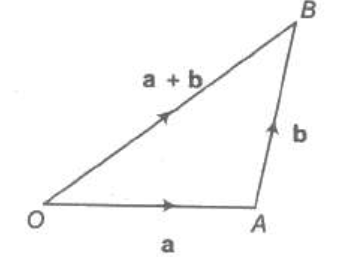(1) Vector addition is commutative i.e. $\vec{A} + \vec{B}= \vec{B} + \vec{A}$
(2) Vector addition is associative i.e. $\vec{A} + \vec{B} + \vec{C}=(\vec{A}+ \vec{B})+\vec{C}=\vec{A}+(\vec{B}+\vec{C})=(\vec{A}+\vec{C})+ \vec{B}$
(3) $\vec{A} + 0= \vec{A}$
(4) $\vec{A} + (- \vec{A})=0$

## Subtraction of vectors

Let a and b be any two vectors.
Now $\vec{a}- \vec{b} = \vec{a} + (- \vec{b})$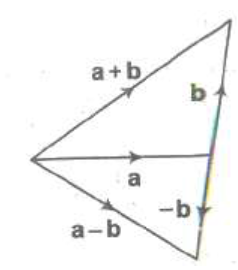So we will first reverse the direction of vector b and then follow the vector addition process
From the terminal point of a, vector -b is drawn. Then, the vector from the initial point  of a to the terminal point B of -b is called the sum of vectors a and -b and is denoted by a - b.

Another method to find subtraction of vectors would be
Let draw vector a and vector b from the same initial point. And then draw the line from end point of vector b to vector a.This will  give a-b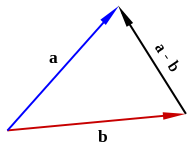## Scalar multiplication of vectors

When we multiply any vector A with any scalar quantity 'n' then it's direction remains unchanged and magnitude gets multiplied by 'n'. Thus, n(A) = nA
Scalar multiplication of vectors is distributive i.e.,
n(A + B) = nA +nB

Important Properties
(i) $|k \vec{a}| = |k| |\vec{a}|$
(ii) $k \vec{0} = \vec{0}$
(iii) $m (-\vec{a}) =- m \vec{a} = - (m \vec{a})$
(iv) $(-m) (-\vec{a}) = m \vec{a}$
(v) $m (n \vec{a}) = mn \vec {a} = n(m \vec{a})$
(vi) $(m + n) \vec{a} = m \vec{a}+ n \vec{a}$
(vii) $m (\vec{a} + \vec{b}) = m \vec{a} + m \vec{b}$

Important Note :Addition and subtraction of scalars make sense only for quantities with same units. However, you can multiply and divide scalars of different units

## Components of the vector

Let a and b be any two non-zero vectors in a plane with different directions and let A be another vector in the same plane A can be expressed as a sum of two vectors ? one obtained by multiplying a by a real number and the other obtained by multiplying b by another real number. To see this, let O and P be the tail and head of the vector A. Then, through O, draw a straight line parallel to a, and through P, a straight line parallel to b. Let them intersect at Q. Then, we have
A = OP = OQ + QP
But since OQ is parallel to a, and QP is parallel to b, we can write :
OQ = λ a, and QP = μ b ( where λ and μ are real numbers.)
Therefore, A = λ a + μ b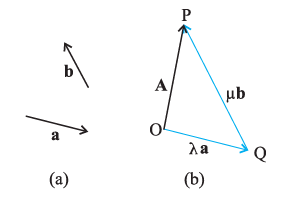We say that A has been resolved into two component vectors λ a and μb along a and b respectively.
Similarly We can  represent any vector in rectangular components form. Let us assume an xyz coordinate plane and unit vector $\hat{i}$,$\hat{j}$ and $\hat{k}$ are defined across x,y,z respectively
Then we can represent any vector in the components forms like
$\vec{r}= x\hat{i} +y\hat{j}+ z\hat{k}$
Important takeaways
• x,y and z are scalar components of vector r
• $x\hat{i}$,$y\hat{j}$,$z\hat{k}$ are called the vector components
• x,y,z are termed as rectangular components
• Length of vector or magnitude of the vector is defined as
• $\sqrt {x^2 + y^2 +z^2}$
• x,y,z are called the direction ratio of vector r
• In case it is given l,m,n are direction cosines of a vector then
li+mj+nk=(cosθx) i +(cosθy) j+(cosθz) k    is the unit vector in the direction of the vector and θx,θy,θz    are the angles which the vector makes with x,y and z axis

Addition,subtraction and multiplication,equality in component form can be expressed
In component form addition of two vectors is
$\vec{C}=\vec{A}+ \vec{B}$
$\vec{C} = (A_x+ B_x)\hat{i} + (A_y+ B_y)\hat{j} + (A_z+ B_z)\hat{k}$
Where, $\vec{A} = (A_x, A_y, A_z)$ and $\vec{B} = (B_x, B_y, B_z)$
Thus in component form resultant vector C becomes,
$C_x = A_x+ B_x$ : $C_y = A_y+ B_y$ : $C_z = A_z+ B_z$

In component form subtraction of two vectors is
$\vec{D}=\vec{A} - \vec{B}$
$\vec{D} = (A_x- B_x)\hat{i}+ (A_y- B_y)\hat{j} + (A_z- B_z)\hat{k}$
where, $\vec{A} = (A_x, A_y, A_z)$ and $\vec{B} = (B_x, B_y, B_z)$
Thus in component form resultant vector D becomes,
$D_x = A_x - B_x$ , $D_y = A_y- B_y$ , $D_z = A_z- B_z$

Equality of vector in components form
$\vec{A}=\vec{B}$
$A_x\hat{i} +A_y\hat{j}+A_z\hat{k}= B_x\hat{i}+B_y\hat{j}+B_z\hat{k}$
$A_x=B_x$
$A_y=B_y$
$A_z=B_z$

Multiplication of scalar by vector in components form
$\vec{A}=k\vec{B}$
$=K(B_x\hat{i}+B_y\hat{j}+B_z\hat{k})$
$=(kB_x)\hat{i} + (kB_y)\hat{j}+ (kB_z)\hat{k}$

## Scalar Product

The scalar product or dot product of any two vectors $\vec{A}$ and $\vec{B}$, denoted as $\vec{A}.\vec{B}$ (read A dot B) is defined as
$\vec{A}.\vec{B} = A B cos \theta$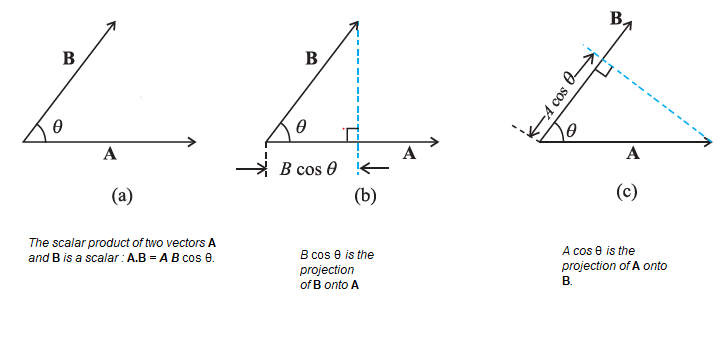Scalar Product follows commutative law and distributive law
$\vec{A}.\vec{B}= \vec{B}.\vec{A}$
$\vec{A}.(\vec{B}+\vec{C}) =\vec{A}.\vec{B} + \vec{A}.\vec{C}$
$\vec{A}.\lambda B=\lambda \vec{A}.\vec{B}$
For Unit vectors
$\hat{i} . \hat{i} = \hat{j}. \hat{j} = \hat{k} .\hat{k} =1$
$\hat{i} . \hat{j} = \hat{j}. \hat{k} = \hat{k}. \hat{i} = 0$
if $\vec{A}=A_x \hat{i} +A_y \hat{j}+A_z \hat{k}$
and $\vec{B}=B_x\hat{i}+B_y\hat{j}+B_z\hat{k}$
then
$\vec{A}.\vec{B}=A_xB_x + A_yB_y+ A_z B_z$
Also
$A.A= A^2 = A_x^2 + A_y^2 + A_z^2$

## Cross product of vectors

• Cross product or vector product of two vectors $\vec{A}$and $\vec{B}$ is defined as
$\vec{A} \text{x} \vec{B} = |\vec{A}| |\vec{B}| sin \theta \hat{n}$
where $\hat{n}$ is the unit vector pointing in the direction perpendicular to the plane of both A and B. Result of vector product is also a vector quantity.
• Cross product is distributive i.e., $\vec{A} \text{x} ( \vec{B} + \vec{C}) = (\vec{A} \text{x} \vec{B}) + (\vec{A} \text{x} \vec{C})$ but not commutative
• The cross product of two parallel vectors is zero.
• Component of any vector is the projection of that vector along the three coordinate axes X, Y, Z.
$\vec{A} \text{x} \vec{B} = (A_x\hat{i} + A_y\hat{j} + A_z\hat{k}) x (B_x\hat{i} + B_y\hat{j} + B_z\hat{k})$
$= (A_yB_z - A_zB_y)\hat{i} + (A_zB_x - A_xB_z)\hat{j} + ( A_xB_y - A_yB_x)\hat{k}$.
•  Cross product of two vectors is itself a vector.
•  To calculate the cross product, form the determinant whose first row is x, y, z, whose second row is A (in component form), and whose third row is B.

## Solved examples

Question 1
We have unit vector $\hat{i}$ point towards the East and the unit vector point $\hat{j}$ toward the North. A person makes displacement $\vec{A}=2\hat{i} + 2\hat{j}$ and $\vec{B}=\hat{i} - 5\hat{j}$. In which approximate direction does the resultant displacement $\vec{C}= \vec{A} + \vec{B}$ points to?
Solution
Resultant Displacement is given by
$\vec{C}= \vec{A} + \vec{B}$
$\vec{C}=2\hat{i} + 2\hat{j} + \hat{i} - 5\hat{j}= 3 \hat{i} - 3\hat{j}$
If we try to position this vector in the plane, then it will clear points in South of east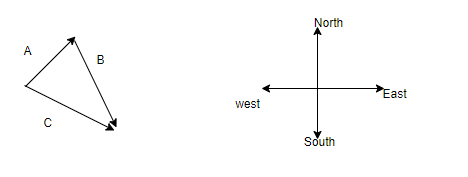Question 2
Given the vectors
$\vec{X}=\hat{i} + \hat{j}$
$\vec{Y}=\hat{i} + 4\hat{j}$
(a) draw the vector sum $\vec{R}= \vec{X} + \vec{Y}$
(b) draw the vector difference $\vec{S}= \vec{X} - \vec{Y}$
(c) Calculate $\vec{R}$ and $\vec{S}$ in terms of unit vectors
Solution
(a) and (b) is shown as below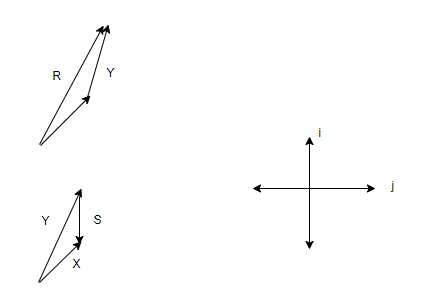(c)$\vec{R}= \vec{X} + \vec{Y}$
$\vec{R}=\hat{i} + \hat{j} + \hat{i} + 4\hat{j}= 2\hat{i} +5 \hat{j}$
$\vec{S}= \vec{X} - \vec{Y}$
$\vec{S} \hat{i} + \hat{j} -( \hat{i} + 4\hat{j})=-3 \hat{j}$

## Concept Map's for Vector addition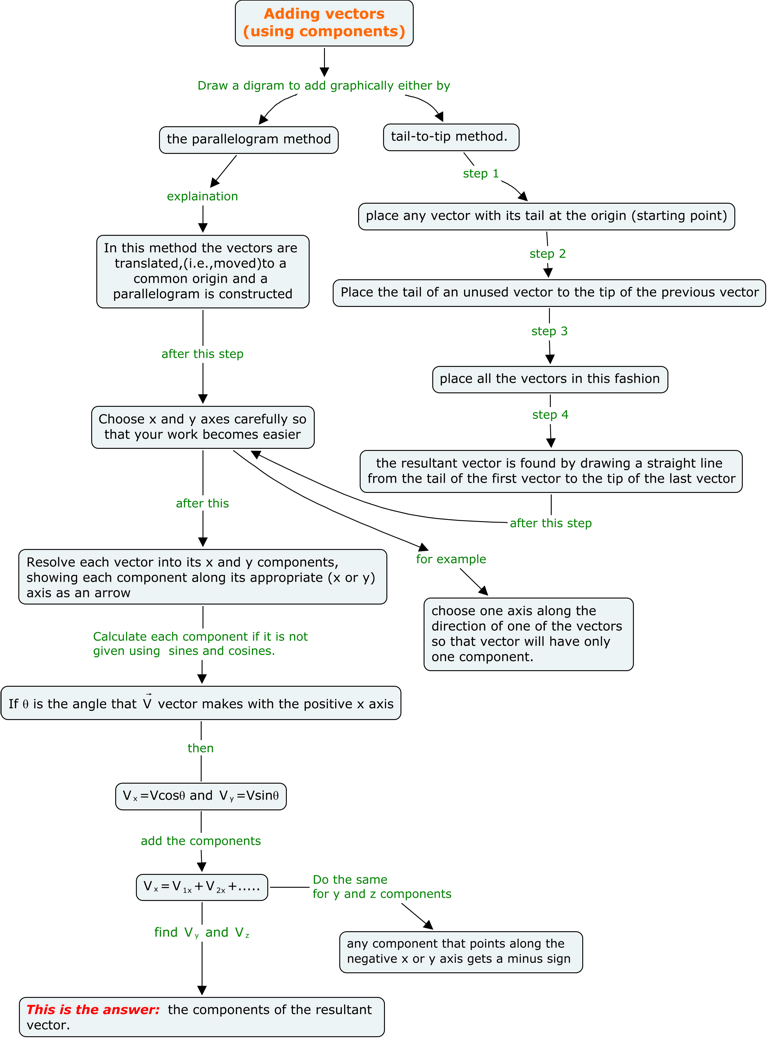## Summary

Here is the summary on Vectors in Physics
• Vectors can be added or subtracted using Parallelogram methods
• Dot Product of vectors is a scalar quantity
• Cross Product of vectors in a Vector quantity
• We can add, subtract, multiplly using the vector components method